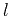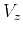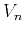Nonhyperbolic reflection moveout of-waves: An overview and comparison of reasonsNext: VERTICAL HETEROGENEITY Up: Fomel & Grechka: Nonhyperbolic Previous: WEAK ANISOTROPY APPROXIMATION for

# HORIZONTAL REFLECTOR BENEATH A HOMOGENEOUS VTI MEDIUM

To exemplify the use of weak anisotropy, let us consider the simplest model of a homogeneous VTI medium above a horizontal reflector. For an isotropic medium, the reflection traveltime curve is an exact hyperbola, as follows directly from the Pythagorean theorem (Figure 2)(9)

wheredenotes the depth of reflector,is the offset,is the zero-offset traveltime, andis the isotropic velocity. For a homogeneous VTI medium, the velocityin equation (9) is replaced by the angle-dependent group velocity. This replacement leads to the exact traveltimes if no approximation for the group velocity is used, since the ray trajectories in homogeneous VTI media remain straight, and the reflection point does not move. We can also obtain an approximate traveltime using the approximate velocitydefined by equations (1) or (5), where the ray angleis given by(10)

Substituting equation (10) into (5) and linearizing the expression(11)

with respect to the anisotropic parametersand, we arrive at the three-parameter nonhyperbolic approximation (Tsvankin and Thomsen, 1994)(12)

where the normal-moveout velocityis defined by equation (4). At small offsets, the influence of the parameteris negligible, and the traveltime curve is nearly hyperbolic. At large offsets, the third term in equation (12) has a clear influence on the traveltime behavior. The Taylor series expansion of equation (12) in the vicinity of the vertical zero-offset ray has the form(13)

When the offsetapproaches infinity, the traveltime approximately satisfies an intuitively reasonable relationship(14)

where the horizontal velocityis defined by equation (3). Approximation (12) is analogous, within the weak-anisotropy assumption, to the skewed hyperbola'' equation (Byun et al., 1989) which uses the three velocities,, andas the parameters of the approximation:(15)

The accuracy of equation (12), which usually lies within 1% error up to offsets twice as large as reflector depth, can be further improved at any finite offset by modifying the denominator of the third term (Grechka and Tsvankin, 1998; Alkhalifah and Tsvankin, 1995).nmoone
Figure 2.
Reflected rays in a homogeneous VTI layer above a horizontal reflector (a scheme).Muir and Dellinger (1985) suggested a different nonhyperbolic moveout approximation in the form(16)

whereis the dimensionless parameter of anellipticity. At large offsets, equation (16) approaches(17)

Comparing equations (14) and (17), we can establish the correspondence(18)

Taking this equality into account, we see that equation (16) is approximately equivalent to equation (12) in the sense that their difference has the order of.Nonhyperbolic reflection moveout of-waves: An overview and comparison of reasonsNext: VERTICAL HETEROGENEITY Up: Fomel & Grechka: Nonhyperbolic Previous: WEAK ANISOTROPY APPROXIMATION for

2014-01-27